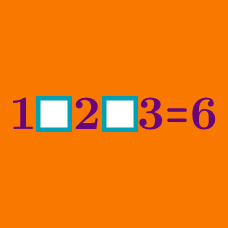Logic

# Filling in parenthesis (only) - Operator Search

Can we add parenthesis such that the expression is equal to 24?

$1 + 2 + 3 \times 4$

$2 \div 2 \div 2 \div 2$

The above expression can be parenthesized in various ways. Which of the following gives the largest value?

$9 - 7 \times 5 - 3$

The above expression can be parenthesized in various ways. Which of the following gives the smallest value?

$3 \div 4 - 5 \times 6$

The above expression can be parenthesized in various ways. Which of the following gives an integer value?

Can we add parenthesis such that the expression is equal to 17?

$9 + 8 \times 7 - 6$

×